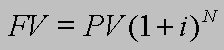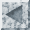## Compound Interest Formula

FV=PV(1+i)^N## Annuity Formula

FV=PMT(1+i)((1+i)^N - 1)/i```     where
PV = present value
FV = future value
PMT = payment  per period
i = interest rate in percent per period
N = number of periods
```

#### Example

```          PMT = \$200 per month
i = 15% per year = 1.25% per month = 0.0125
N = 30 years = 360 months```Application of the formula. Hits